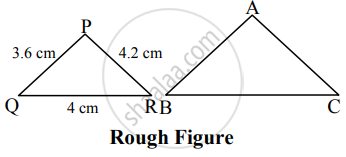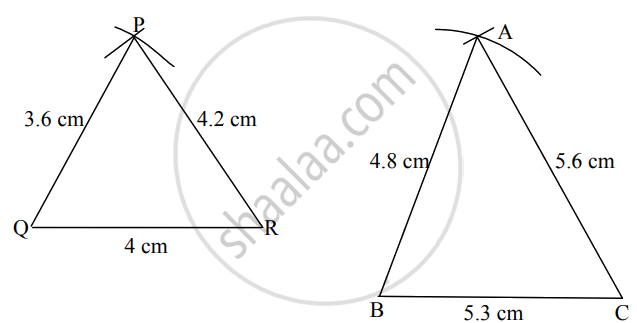# ΔPQR ~ ΔABC. In ΔPQR, PQ = 3.6cm, QR = 4 cm, PR = 4.2 cm. Ratio of the corresponding sides of triangle is 3 : 4, then construct ΔPQR and ΔABC - Geometry Mathematics 2

Diagram

ΔPQR ~ ΔABC. In ΔPQR, PQ = 3.6cm, QR = 4 cm, PR = 4.2 cm. Ratio of the corresponding sides of triangle is 3 : 4, then construct ΔPQR and ΔABC

#### SolutionAnalysis: ∆PQR ∼ ∆ABC

∴ "PQ"/"AB" = "QR"/"BC" = "PR"/"AC"  .....[Corresponding sides of similar triangles]

∴ 3.6/"AB" = 4/"BC" =4.2/"AC" = 3/4   ......[Given]

 ∴ 3.6/"AB" = 3/4 ∴ AB = (3.6 xx 4)/3 ∴ AB = 1.2 × 4 ∴ AB = 4.8 cm 4/"BC" = 3/4 ∴ BC = (4 xx 4)/3 ∴ BC = 16/3 ∴ BC = 5.3 cm (approx) 4.2/"AC" = 3/4 ∴ AC = (4.2 xx 4)/3 ∴ AC = 1.4 × 4 ∴ AC = 5.6 cmSteps of Construction:

 ∆PQR ∆ABC i. Draw seg QR of 4 cm Draw seg BC of 5.3 cm ii. Taking 3.6 cm and 4.2 cm distances on compass draw two arcs from Q and R respectively Taking 4.8 cm and 5.6 cm distance on compass draw two arcs from point B and C respectively. iii. Name the point of intersection as P. Name the point of intersection as A.
Is there an error in this question or solution?
Chapter 4: Geometric Constructions - Q.3 (B)

Share